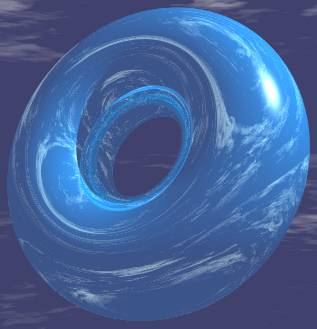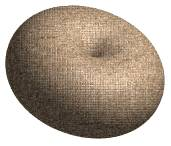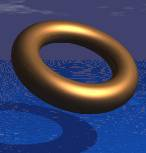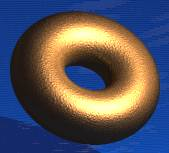# Torus

Go to Surface Area or Volume.

Torus Facts

Notice these interesting things:• It can be made by revolving a
by a bigger circle (radius R).
• It has no edges or vertices
• It is not a polyhedronTorus in the Sky
.
The Torus is such a beautiful solid,
this one would be fun at the beach !
images/poly-gl.js?mode=torus

## Surface AreaSurface Area = (2πR) × (2πr) = 4 × π2 × R × r

### Example: r = 3 and R = 7

Surface Area = 4 × π2 × R × r
= 4 × π2 × 7 × 3
= 4 × π2 × 21
= 84 × π2
≈ 829

The formula is often written in this shorter way:

Surface Area = 4π2Rr

## Volume

 Volume = (2πR) × (πr2) = 2 × π2 × R × r2

### Example: r = 3 and R = 7

Volume = 2 × π2 × R × r2
= 2 × π2 × 7 × 32
= 2 × π2 × 7 × 9
= 126 π2
≈ 1244

The formula is often written in this shorter way:

Volume = 2π2 Rr2

Note: Area and volume formulas only work when the torus has a hole!

## Like a Cylinder

Volume: the volume is the same as if we "unfolded" a torus into a cylinder (of length 2πR):As we unfold it, what gets lost from the outer part of the torus is perfectly balanced by what gets gained in the inner part.

Surface Area: the same is true for the surface area, not including the cylinder's bases.And did you know that Torus was the Latin word for a cushion?

(This is not a real roman cushion, just an illustration I made)

The Volume and Area calculations will not work with this cushion because there is no hole.

When we have more than one torus they are called tori

## More Torus Images

As the small radius (r) gets larger and larger, the torus goes from looking like a Tire to a Donut:3391, 3392, 3393, 3394, 3395, 3396, 3397, 3398, 7656, 7657